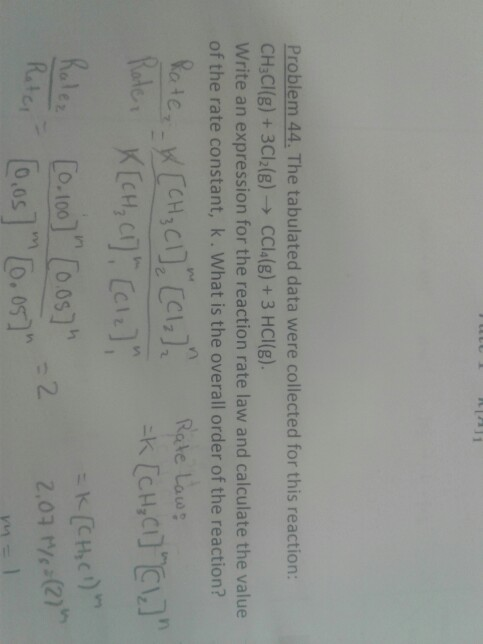# Question & Answer: The tabulated data were collected for this reaction: CH_3Cl(g) + 3Cl_2(g) rightarrow…..The tabulated data were collected for this reaction: CH_3Cl(g) + 3Cl_2(g) rightarrow CCl_4(g) + 3 HCl(g). Write an expression for the reaction rate law and calculate the value of the rate constant, k. What is the overall order of the reaction?